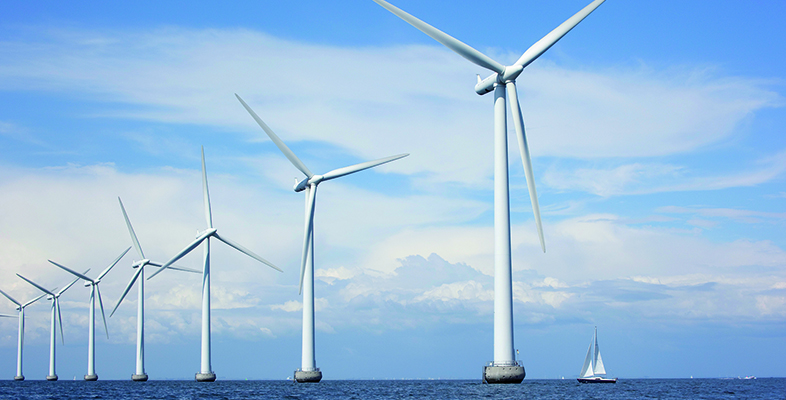Energy in buildings

This free course is available to start right now. Review the full course description and key learning outcomes and create an account and enrol if you want a free statement of participation.

Free course

# 3.5 Heating systems and CO2 emissions

In assessing any particular building we are likely to be interested in its total fuel consumption and its CO2 emissions. The previous sections have given some guidance on estimating the likely net space heating demand. Adding an allowance for water heating, the total annual fuel use for heating can thus be calculated as:

• annual fuel use = annual heat demand / heating system thermal efficiency

Not all heating fuels produce the same amount of CO2 for a given amount of heat produced. Table 11 gives the emission factors for the main UK heating fuels. Note that natural gas is considerably better than electricity and coal. Electricity is particularly bad because of the large heat losses that take place at conventional power stations.

## Table 11 CO2 emission factors for heating fuels

Emissions CO2 /

kg kWh–1

Natural gas 0.22
Liquefied petroleum gas (LPG) 0.24
Heating oil (gas oil) 0.30
House coal 0.39
Electricity 0.52
(Source: BRE, 2012)

Using these figures and likely heating system efficiencies we can calculate the total annual CO2 production.

As described at the beginning of Section 3 the thermal efficiency of a heating system = useful heat output / fuel input.

So annual fuel use = useful heat demand / thermal efficiency

annual CO2 production = annual fuel use × CO2 emission factor

Consider, for example, a house with a total estimated net space heating demand of 10 000 kWh yr–1 plus a water heating demand of 5000 kWh yr–1.

What are its likely annual CO2 emissions if it is heated (a) by a condensing natural gas boiler with a thermal efficiency of 90% or (b) using off-peak storage heating with an assumed efficiency of 100%?

• a.Using a condensing gas boiler:
• total annual fuel use = (10 000 + 5000)/0.90 = 16 667 kWh yr–1
• CO2 emissions = 16 667 × 0.22 = 3667 kg yr–1

• b.Using off-peak electric storage heaters:
• total annual fuel use = (10 000 + 5000) / 1.0 = 15 000 kWh yr–1
• annual CO2 emissions = 15 000 × 0.52 = 7800 kg yr–1

Thus the electric heating option produces well over twice the CO2 emissions of the gas option.

## Activity 14

Supposing the house described above is in a rural location and doesn’t have access to mains natural gas. What are the likely annual CO2 emissions if it is heated:

• a.by a condensing liquefied petroleum gas (LPG) boiler with a thermal efficiency of 93%?
• b.by a ground source heat pump with a system performance factor of 2.80?

Which has the lower annual CO2 emissions?

### Answer

• a.Using a LPG condensing gas boiler:

total annual fuel use = (10 000 + 5000) / 0.93 = 16 129 kWh yr-1

From Table 11, the emission factor for LPG is 0.24 kg CO2 per kWh

So annual CO2 emissions = 16 129 × 0.24 = 3871 kg yr-1

• b.Using a heat pump:

The useful heat produced by the heat pump = SPF × electricity used.

So electricity used = useful heat / SPF

= (10 000 + 5000) / 2.80 = 5357 kWh yr-1

Annual CO2 emissions = 5357 × 0.52 = 2786 kg yr-1

The heat pump wins!

T313_1

### Take your learning further

Making the decision to study can be a big step, which is why you'll want a trusted University. The Open University has 50 years’ experience delivering flexible learning and 170,000 students are studying with us right now. Take a look at all Open University courses.

If you are new to university level study, find out more about the types of qualifications we offer, including our entry level Access courses and Certificates.

Not ready for University study then browse over 900 free courses on OpenLearn and sign up to our newsletter to hear about new free courses as they are released.

Every year, thousands of students decide to study with The Open University. With over 120 qualifications, we’ve got the right course for you.

Request an Open University prospectus